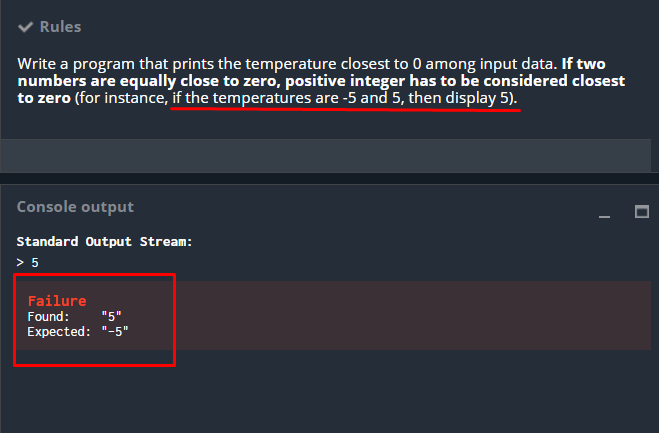Temperatures: Failure Found: 5 Expected: -5

#1

Hi all,

Is this normal ?this is my code

``````import sys
import math

# Auto-generated code below aims at helping you parse
# the standard input according to the problem statement.
B = 5526
n = int(input())  # the number of temperatures to analyse
for i in input().split():
# t: a temperature expressed as an integer ranging from -273 to 5526
t = int(i)
if abs(t) < B:

B = abs(t)

# Write an action using print
# To debug: print("Debug messages...", file=sys.stderr)

print(B)``````

#2

You shall only print the positive value, if you have both the positive and negative with the same distance to 0.
In that testcase where you fail, there are only negative inputs.

#3

Yeap, figured it out at a later time. I should refrain myself from posting so easily. Thank you for following up.